Processing ......FreeComputerBooks.com Links to Free Computer, Mathematics, Technical Books all over the World

Notes on Convex sets, Polytopes, Polyhedra, Combinatorial Topology, Voronoi Diagrams and Delaunay Triangulations
Top Free Java Books 🌠 - 100% Free or Open Source!
• Title Notes on Convex sets, Polytopes, Polyhedra, Combinatorial Topology, Voronoi Diagrams and Delaunay Triangulations
• Authors Jean Gallier
• Publisher: Arxiv.org
• Paperback: N/A
• eBook: PDF (195 pages)
• Language: English
• ISBN-10: N/A
• ISBN-13: N/ANotes on Convex sets, Polytopes, Polyhedra, Combinatorial Topology Jean Gallier

Book Description

This book may be viewed as a tutorial and a set of notes on convex sets, polytopes, polyhedra, combinatorial topology, Voronoi Diagrams and Delaunay Triangulations. It is intended for a broad audience of mathematically inclined readers.

• Jean Gallier is a professor at Computer and Information Science Department, School of Engineering and Applied Science, University of Pennsylvania.
Reviews and Rating: Related Book Categories: Read and Download Links:Similar Books:
•Convex Bodies and Algebraic Geometry: Theory of Toric Varieties

This book is an accessible introduction to current algebraic geometry of toric varieties gives new insight into continued fractions as well as their higher-dimensional analogues, the isoperimetric problem and other questions on convex bodies.

•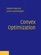Convex Optimization (Stephen Boyd, et al.)

On recognizing convex optimization problems and then finding the most appropriate technique for solving them. It contains many worked examples in fields such as engineering, computer science, mathematics, statistics, finance, and economics.

•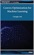Convex Optimization for Machine Learning (Changho Suh)

This book covers an introduction to convex optimization, one of the powerful and tractable optimization problems that can be efficiently solved on a computer. The goal of the book is to help develop a sense of what convex optimization is, and how it can be used.

•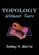Topology without Tears (Sidney A. Morris)

General topology is the branch of topology dealing with the basic set-theoretic definitions and constructions used in topology. The aim of this is to provide a thorough grouding of general topology. It offers an ideal introduction to the fundamentals of topology.

•Topological Groups: Yesterday, Today, Tomorrow (Sidney A. Morris)

In 1900, David Hilbert asked whether each locally euclidean topological group admits a Lie group structure. This was the fifth of his famous 23 questions which foreshadowed much of the mathematical creativity of the twentieth century.

•Topology: A Categorical Approach (Tai-Danae Bradley, et al)

A graduate-level textbook that presents basic topology from the perspective of category theory. Many graduate students are familiar with the ideas of point-set topology and they are ready to learn something new about them.

•Manifolds - Current Research Areas (Paul Bracken)

This book cover a number of subjects which will be of interest to workers in these areas. It is hoped that the papers here will be able to provide a useful resource for researchers with regard to current fields of research in this important area.

•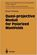Quasi-projective Moduli for Polarized Manifolds (Eckart Viehweg)

This book discusses two subjects of quite different nature: Construction methods for quotients of quasi-projective schemes by group actions or by equivalence relations and properties of direct images of certain sheaves under smooth morphisms.

•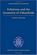Foliations and the Geometry of 3-Manifolds (Danny Calegari)

This book is to expose the "pseudo-Anosov" theory of foliations of 3-manifolds, which generalizes Thurston's theory of surface automorphisms, and reveals an intimate connection between dynamics, geometry and topology in 3 dimensions.

•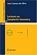Lectures on Symplectic Geometry (Ana Cannas da Silva)

The goal of these notes is to provide a fast introduction to symplectic geometry for graduate students with some knowledge of differential geometry, de Rham theory and classical Lie groups.

•Fractal Geometry: Mathematical Foundations and Applications

This book has become a seminal text on the mathematics of Fractals. It introduces the general mathematical theory and applications of fractals in a way that is accessible to students from a wide range of disciplines.

Book Categories
 :All CategoriesTop Free BooksRecent BooksMiscellaneous BooksComputer EngineeringComputer LanguagesComputer ScienceData Science/DatabasesJava and Java EE (J2EE)Linux and UnixMathematicsMicrosoft and .NETMobile ComputingNetworking and CommunicationsSoftware EngineeringSpecial TopicsWeb Programming
Other Categories